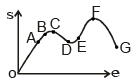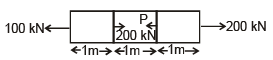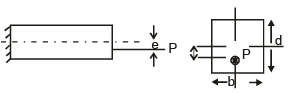# Test: Strength of Materials - 1

## 25 Questions MCQ Test Mechanical Engineering SSC JE (Technical) | Test: Strength of Materials - 1

Description
Attempt Test: Strength of Materials - 1 | 25 questions in 50 minutes | Mock test for Mechanical Engineering preparation | Free important questions MCQ to study Mechanical Engineering SSC JE (Technical) for Mechanical Engineering Exam | Download free PDF with solutions
QUESTION: 1

Solution:
QUESTION: 2

Solution:
QUESTION: 3

###The above graph is relation between stress and strain for:

Solution:
QUESTION: 4In the above graph which of the following represents ultimate tensile strength.

Solution:
QUESTION: 5

In a material is under cyclic load we used endurance strength as designing criterion. The failure due to such loading is because of :

Solution:
QUESTION: 6

Modulus of toughness is area under –

Solution:
QUESTION: 7

If the percentage of carbon in steel increases which of the following properties will increase
i. Ductility
ii. Brittleness
iii. Strength
iv. Toughness
v. Hardness

Solution:
QUESTION: 8

A square steel bar of 50 mm side and 5m length absorbs a strain energy of 100 joule when stretched upto its elastic limit. The modulus of resiliance of the bar is :

Solution:
QUESTION: 9

A square column has a side of 10m. What is the area of its core.

Solution:
QUESTION: 10

The total elongation of the shown bar is A = 5m2 E = 200 GPaSolution:
QUESTION: 11

For the shown figure when will be the stress at point A is tensile in nature, whenSolution:
QUESTION: 12

The relation ship b/w shear angle (f) and angle of twist(q) can be given as.

Solution:
QUESTION: 13

The shear stress distribution for a E section will be like.

Solution:
QUESTION: 14

A ductile material will fail because of -

Solution:
QUESTION: 15

The dimension of strain is

Solution:
QUESTION: 16

The dimension of young's modulus (E) is

Solution:
QUESTION: 17

For what value of poision ratio (m) Bulk modulus and shear modulus can be equal.

Solution:
QUESTION: 18

A shaft made of ductile material is subjected to pure torsion. In which of the following direction will fracture occur.

Solution:
QUESTION: 19

Poisions' Ratio is ratio between

Solution:
QUESTION: 20

A shaft of shear modulus 80 GPa, polar moment of inertia 80 mm4 and length 1m. What is its torsional stiffness.

Solution:
QUESTION: 21

If young's modulus is equal to bulk modulus, the value of poisions ratio is

Solution:
QUESTION: 22

The relationship b/w G,K,E can be given as,

Solution:
QUESTION: 23

The B.M.D for a simply supported beam with point load will be

Solution:
QUESTION: 24

Given the curve of bending moment is y3 - 3y2.The curve of shear force is

Solution:
QUESTION: 25

Radius of gyration for a square cross section of area a2 is

Solution:Use Code STAYHOME200 and get INR 200 additional OFF Use Coupon Code